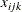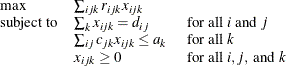### Example 5.3 Model Construction

This example uses PROC OPTMODEL features to simplify the construction of a mathematically formulated model. The model is based on the example An Assignment Problem in Chapter 5: The LP Procedure in SAS/OR 12.3 User's Guide: Mathematical Programming Legacy Procedures. A single invocation of PROC OPTMODEL replaces several steps in the PROC LP statements.

The model assigns production of various grades of cloth to a set of machines in order to maximize profit while meeting customer demand. Each machine has different capacities to produce the various grades of cloth. (See the PROC LP example An Assignment Problem for more details.) The mathematical formulation, whererepresents the amount of cloth of gradeto produce on machinefor customer, follows:The OBJECT, DEMAND, and RESOURCE data sets are the same as in the PROC LP example. A new data set, GRADE, is added to help separate the data from the model.

title 'An Assignment Problem';

data grade(drop=i);
do i = 1 to 6;
grade = 'grade'||put(i,1.);
output;
end;
run;

data object;
input machine customer
grade1 grade2 grade3 grade4 grade5 grade6;
datalines;
1 1 102 140 105 105 125 148
1 2 115 133 118 118 143 166
1 3  70 108  83  83  88  86
1 4  79 117  87  87 107 105
1 5  77 115  90  90 105 148
2 1 123 150 125 124 154   .
2 2 130 157 132 131 166   .
2 3 103 130 115 114 129   .
2 4 101 128 108 107 137   .
2 5 118 145 130 129 154   .
3 1  83   .   .  97 122 147
3 2 119   .   . 133 163 180
3 3  67   .   .  91 101 101
3 4  85   .   . 104 129 129
3 5  90   .   . 114 134 179
4 1 108 121  79   . 112 132
4 2 121 132  92   . 130 150
4 3  78  91  59   .  77  72
4 4 100 113  76   . 109 104
4 5  96 109  77   . 105 145
;

data demand;
input customer
grade1 grade2 grade3 grade4 grade5 grade6;
datalines;
1 100 100 150  150  175  250
2 300 125 300  275  310  325
3 400   0 400  500  340    0
4 250   0 750  750    0    0
5   0 600 300    0  210  360
;

data resource;
input machine
grade1 grade2 grade3 grade4 grade5 grade6 avail;
datalines;
1 .250 .275 .300  .350  .310  .295  744
2 .300 .300 .305  .315  .320  .     244
3 .350 .    .     .320  .315  .300  790
4 .280 .275 .260  .     .250  .295  672
;


The following PROC OPTMODEL statements read the data sets, build the linear programming model, solve the model, and output the optimal solution to a SAS data set called SOLUTION:

proc optmodel;
/* declare index sets */
set CUSTOMERS;
set <str> GRADES;
set MACHINES;

/* declare parameters */
num return {CUSTOMERS, GRADES, MACHINES} init 0;
num demand {CUSTOMERS, GRADES};
num cost {GRADES, MACHINES} init 0;
num avail {MACHINES};

/* read the set of grades */
read data grade into GRADES=[grade];

/* read the set of customers and their demands */
read data demand
into CUSTOMERS=[customer]
{j in GRADES} <demand[customer,j]=col(j)>;

/* read the set of machines, costs, and availability */
read data resource nomiss
into MACHINES=[machine]
{j in GRADES} <cost[j,machine]=col(j)>
avail;

/* read objective data */
read data object nomiss
into [machine customer]
{j in GRADES} <return[customer,j,machine]=col(j)>;

/* declare the model */
var AmountProduced {CUSTOMERS, GRADES, MACHINES} >= 0;
max TotalReturn = sum {i in CUSTOMERS, j in GRADES, k in MACHINES}
return[i,j,k] * AmountProduced[i,j,k];
con req_demand {i in CUSTOMERS, j in GRADES}:
sum {k in MACHINES} AmountProduced[i,j,k] = demand[i,j];
con req_avail {k in MACHINES}:
sum {i in CUSTOMERS, j in GRADES}
cost[j,k] * AmountProduced[i,j,k] <= avail[k];

/* call the solver and save the results */
solve;
create data solution
from [customer grade machine] = {i in CUSTOMERS, j in GRADES,
k in MACHINES: AmountProduced[i,j,k].sol ne 0}
amount=AmountProduced;

/* print optimal solution */
print AmountProduced;
quit;


The statements use both numeric (NUM) and character (STR) index sets, which are populated from the corresponding data set variables in the READ DATA statements. The OPTMODEL parameters can be either single-dimensional (AVAIL) or multiple-dimensional (COST, DEMAND, RETURN). The RETURN and COST parameters are given initial values of 0, and the NOMISS option in the READ DATA statement tells PROC OPTMODEL to read only the nonmissing values from the input data sets. The model declaration is nearly identical to the mathematical formulation. The logical condition AmountProduced[i,j,k].sol ne 0 in the CREATE DATA statement ensures that only the nonzero parts of the solution appear in the SOLUTION data set. In the PROC LP example, the creation of this data set required postprocessing of the PROC LP output data set.

The solver produces the following problem summary and solution summary:

Output 5.3.1: LP Solver Result

 An Assignment Problem

Problem Summary
Objective Sense Maximization
Objective Function TotalReturn
Objective Type Linear

Number of Variables 120
Bounded Above 0
Bounded Below 120
Bounded Below and Above 0
Free 0
Fixed 0

Number of Constraints 34
Linear LE (<=) 4
Linear EQ (=) 30
Linear GE (>=) 0
Linear Range 0

Constraint Coefficients 220

Solution Summary
Solver LP
Algorithm Dual Simplex
Objective Function TotalReturn
Solution Status Optimal
Objective Value 871426.03763

Primal Infeasibility 0
Dual Infeasibility 0
Bound Infeasibility 0

Iterations 60
Presolve Time 0.00
Solution Time 0.00

The SOLUTION data set can be processed by PROC TABULATE as follows to create a compact representation of the solution:

proc tabulate data=solution;
class customer grade machine;
var   amount;
table (machine*customer), (grade*amount=''*sum='');
run;


These statements produce the table shown in Output 5.3.2.

Output 5.3.2: An Assignment Problem

 An Assignment Problem

grade
grade1 grade2 grade3 grade4 grade5 grade6
machine customer . 100.00 150.00 150.00 175.00 250.00
1 1
2 . . 300.00 . . .
3 . . 256.72 210.31 . .
4 . . 750.00 . . .
5 . 92.27 . . . .
2 3 . . 143.28 . 340.00 .
5 . . 300.00 . . .
3 2 . . . 275.00 310.00 325.00
3 . . . 289.69 . .
4 . . . 750.00 . .
5 . . . . 210.00 360.00
4 1 100.00 . . . . .
2 300.00 125.00 . . . .
3 400.00 . . . . .
4 250.00 . . . . .
5 . 507.73 . . . .